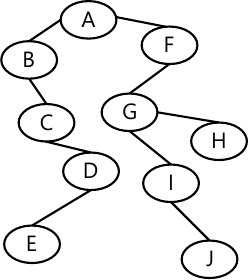MORE IN Data Structures and Applications
VTU Computer Science (Semester 3)
Data Structures and Applications
December 2012
Total marks: --
Total time: --
INSTRUCTIONS
(1) Assume appropriate data and state your reasons
(2) Marks are given to the right of every question
(3) Draw neat diagrams wherever necessary

1 (a) What are pointer variables? How to declare a pointer variable?
5 M
1 (b) What are the various memory allocation techniques? Explain how memory can be dynamically allocated using malloc()?
10 M
1 (c) What is recursion? What are the various types of recursion?
5 M

2 (a) What is the difference between int *a and int a and int *?
6 M
2 (b) What is a structure? How to declare and initialize a structure?
6 M
2 (c) Write a programin C o.read a sparse matrix of integer values and search this matrix for an element specified by the user.
8 M

3 (a) Define stack. List the operation on stack.
8 M
3 (b) Obtain the postfix and prefix expression for (((A+(B-C)*D)E)+F)
6 M
3 (c) What is system stack? How the control is transferred to or from the function with the help of activation record?
6 M

4 (a) What is a linked list? Explain the different types of linked list with diagram.
10 M
4 (b) Write a function to insert a node at front and rear end in a circular linked list. Write down sequence of steps to be followed.
10 M

5 (a) What is a tree? Explain: i) root.node, ii) child, iii) siblings, iv) ancestors using structure representation.
6 M
5 (b) What is a binary tree? How it is represented using array and linklist?
10 M
5 (c) What is a heap? Explain the different types of heap?
4 M

6 (a) What is the binary search tree? Draw the binary serach tree for the following input:
14, 5, 6, 2, 18, 20, 16, 18, -1, 21.
10 M
6 (b) What is a forest? Explain the different method of traversing a tree with following tree;10 M

7 (a) What is priority queue? Explain the various types of priority queues.
8 M
7 (b) Write short notes on:
i) Binomial heaps, ii) Fibonacci heap
6 M
7 (c) What is leftist tree? Explain different types of leftist trees.
6 M

8 (a) What is an AVL tree? Write the algorithm to insert an item into AVL tree.
8 M
8 (b) Write short notes on: i) Red-black tree ii) Spaly trees.
6 M
8 (c) Explain the different types of rotations of an AVL tree.
6 M

More question papers from Data Structures and Applications